Courses

# Test: Oxygen & Sulphur Compounds

## 25 Questions MCQ Test Chemistry Class 12 | Test: Oxygen & Sulphur Compounds

Description
This mock test of Test: Oxygen & Sulphur Compounds for JEE helps you for every JEE entrance exam. This contains 25 Multiple Choice Questions for JEE Test: Oxygen & Sulphur Compounds (mcq) to study with solutions a complete question bank. The solved questions answers in this Test: Oxygen & Sulphur Compounds quiz give you a good mix of easy questions and tough questions. JEE students definitely take this Test: Oxygen & Sulphur Compounds exercise for a better result in the exam. You can find other Test: Oxygen & Sulphur Compounds extra questions, long questions & short questions for JEE on EduRev as well by searching above.
QUESTION: 1

### Direction (Q. Nos. 1-10) This section contains 10 multiple choice questions. Each question has four choices (a), (b), (c) and (d), out of which ONLY ONE is correct. Q.  Which of the following statement is correct about the hydride of group 16 elements?

Solution:

Order of acidic strength, thermal stability and melting points and boiling points are given in correct order.

QUESTION: 2

### The pair that contains mixed oxides is

Solution:

Mixed oxides are made up of two simple oxides like
Pb3O4 (2PbO + PbO2), Fe3O4 (FeO + Fe2O3) , Mn3O4 (2MnO + MnO2

QUESTION: 3

### The acid having a sulp hur atom with a lone pair of electrons on it, is

Solution:

Sulphur having valency 6 do not possess lone pair while the sulphur with valency 4 possess one lone pair.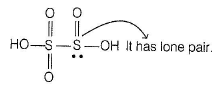QUESTION: 4

Charring of cane sugar by sulphuric acid shows ...... property of the acid.

Solution:

Cone. H2SO4 is a strong dehydrating agent. Many wet gases can be dried by passing them through sulphuric acid. H2SO4 removes water from organic compounds. It is evident by its charring action on carbohydrates.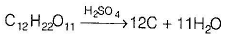QUESTION: 5

Which of the following statements is incorrect?

Solution:

Disulphur dichlor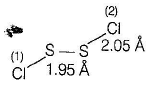The angle between  the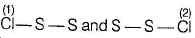planes is 90°.
The structure is referred to as gauche.

QUESTION: 6

xSo2 + yMnO-4 + zH2O → Mn2+ + SO42- + H+ . In this, three values of x, y, z are

Solution: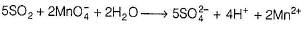QUESTION: 7

Which of the following is wrong statement?

Solution:

The chemical reaction of H2SO4 as a result of its low volatility, strong acidic character, stiong affinity for water and ability to act as an oxidising agent.

QUESTION: 8

Among the following oxyacids of sulphur, which one has more than one oxidation state for sulphur?

Solution:

In thiosulphuric acid, S has both - 2 and + 6 oxidation states.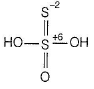QUESTION: 9

In SO3 molecule, 3 oxygen atoms are attached to sulphur atom through double bonds. Hence, the molecules consist 3π-bonds. The types of pi-bonds present in SO3 molecule are

Solution:

In gas phase, SO3 molecule is triangular planar having sp2-hybridisation of the S-atom. There are three sigma bonds formed by sp2-p overlap and three pi-bonds are by pπ-pπ overlap and two pπ-dπ overlap.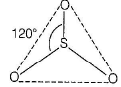QUESTION: 10

Which of the following is known as C aro’s acid?

Solution:

H2SO5 is known as Caro's acid or peroxysuiphuric acid.

QUESTION: 11

Direction (Q. No. 11) This section is based on Statements I and Statements II. Select the correct answer from the codes given below.

Q.

Statement I : In Caro’s acid (H2SO5), the oxidation state of sulphur is found to be +8.

Statement II : In Caro’s acid, sulphur atom is attached to four oxygen atoms directly.

Solution:

The oxidation state of sulphur in caro's acid is +6. The structure of the acid is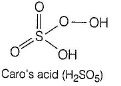The two of the attached O-atoms impart +2 oxidation state each to the sulphur atom and rest two O-atoms (single bonded) imparts +1 oxidation state each to the S-atom. Hence, S-atom has a total of +6 oxidation state

*Multiple options can be correct
QUESTION: 12

Direction (Q. Nos. 12-15) This section contains 4 multiple choice questions. Each question has four choices (a), (b), (c) and (d), out of which ONE or MORE THAN ONE are correct.

Q.

The incorrect statements among the following are

Solution:

In SO2, the oxidation state of S is + 4 , i.e. it can be increased or decreased. Hence, SO2 can act as both oxidant and reductant.

*Multiple options can be correct
QUESTION: 13

The chemical species having sp2-hybridisation for the central atom is

Solution:

SO3 are sp2-hybridised with triangular planar geometry.

*Multiple options can be correct
QUESTION: 14

Which of the following is/are the correct statement(s) about sodium thiosulphate?

Solution:

Sodium thiosulphate is an inorganic compound also used to treat fungal infection of skin diseases.

*Multiple options can be correct
QUESTION: 15

Which pair is isostructural?

Solution:

(i) CO2 and OCS both are sp-hybrid ised and have linear geometry.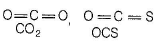(ii)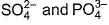both are sp3-hybridised and have tetrahedral geometry.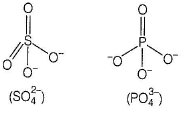QUESTION: 16

Direction (Q. Nos. 16 and 17) This section contains a paragraph, describing theory, experiments, data, etc. Two questions related to the paragraph have been given. Each question has only one correct answer among the four given options (a), (b), (c) and (d).

Passage

Hydrated sodium thiosulphate, Na2S2O3 .5H2O is called hypo. It can be prepared by Spring’s reaction by adding iodine to a mixture of sodium sulphide and sodium sulphite.

Na2S + Na2SO3 + I2 → 2NaI + Na2S2O3 (Spring's reaction)
It is an efflorescent substance and at 215°C, it is completely dehydrated to Na2S2O3. Its reaction with iodine is used fo r the iodometric estimation of oxidising agents like CuSO4, CaOCI2, KMnO4, MnO2, etc., which on reaction with KI liberate I2. It is used in the bleaching industry to destroy excess chlorine on fabrics. It is used in photography as fixer since, it dissolves unexposed AgBr on the film.

Q.

Select the incorrect statement regarding hypo.

Solution:

In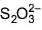, central atom sulphur is sp3-hybridised and it has tetrahedral shape.

QUESTION: 17

Passage

Hydrated sodium thiosulphate, Na2S2O3 .5H2O is called hypo. It can be prepared by Spring’s reaction by adding iodine to a mixture of sodium sulphide and sodium sulphite.

Na2S + Na2SO3 + I2 → 2NaI + Na2S2O3 (Spring's reaction)

It is an efflorescent substance and at 215°C, it is completely dehydrated to Na2S2O3. Its reaction with iodine is used fo r the iodometric estimation of oxidising agents like CuSO4, CaOCI2, KMnO4, MnO2, etc., which on reaction with KI liberate I2. It is used in the bleaching industry to destroy excess chlorine on fabrics. It is used in photography as fixer since, it dissolves unexposed AgBr on the film.

Q.

In Na2S4O6, sulphur has different oxidation states. They are

Solution:

The structure of Na2S4O6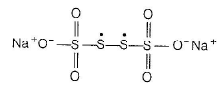In this structure, two central sulphur atoms have zero oxidation number because electron pair forming th e S—S bond remain in the centre. Let the oxidation number of (remaining atoms) S atom be X.
2(+1)+ 6(-2)+ 2x+ 2(0) = 0
For Na for O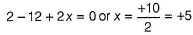∴ Two central S-atoms have zero oxidation state and two terminal S- atoms have +5 oxidation state,

QUESTION: 18

Direction (Q. Nos. 18-20) Choices for the correct combination of elements from Column I and Column II are given as options (a), (b), (c) and (d), out of which one is correct.

Q.

Match the Column I with Column II and mark the correct option from the codes given below.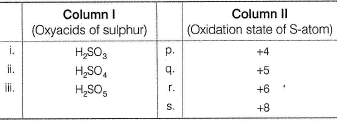Solution:

The oxidation states of sulphur in H2SO3, H2SO4, and H2SO5 are respectively +4, +6 and +6.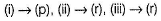QUESTION: 19

Match the Column I with Column II and mark the correct option from the codes given below.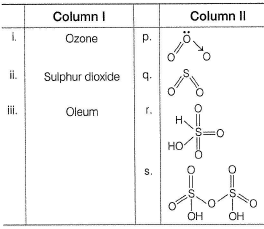Solution: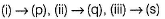QUESTION: 20

Match the Column I with Column II and mark the correct option from the codes given below.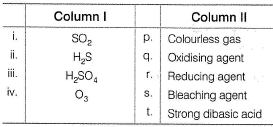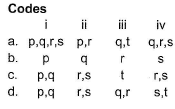Solution: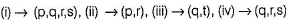*Answer can only contain numeric values
QUESTION: 21

Direction (Q. Nos. 21-25) This section contains 5 questions. When worked out will result in an integer from 0 to 9 (both inclusive).

Q.

The number of S—S bonds in sulphur trioxide trimer (S3O9) are

Solution: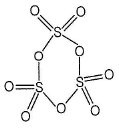*Answer can only contain numeric values
QUESTION: 22

The number of metal sulphides precipitated by passing H2S in acidic medium among
ZnS, PbS, CuS, NiS, MnS, CdS, CoS, HgS, SnS.

Solution:

II group radicals Pb2+, Cu2+ ,Cd2+, Hg2+ ,Sn2+ are precipitated with in acidic medium.
IV group cations Zn2+, Mn2+, CO2+, Ni2+ are precipitated with H2S in alkaline medium.

*Answer can only contain numeric values
QUESTION: 23

The number of chemical species having sp3-hybridisation.
SO3, SO2, SOCI2, SO2CI2, O3, SF2, SF4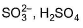Solution:

The four chemical species among the given molecules having sp3-hybridisation of central atom are SO2CI2, SF2,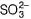and H2SO4

*Answer can only contain numeric values
QUESTION: 24

The number of covalent bonds present in H2SO5.

Solution: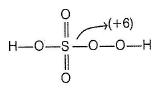H2SO5 (peroxomonosulphuric acid)
It has nine covalent bonds.

*Answer can only contain numeric values
QUESTION: 25

Number of molecules having non-zero dipole moment in
O3, SO3, SF4, SF6, H2S, CS2, SO2, H2O and H2O2.

Solution:

SO3, SF6 and CS2 have zero dipole moment because of symmetry in the structure.

The remaining 6 have dipole moments.

Hence 6 is the correct answer.